# how this result appear?

Status
Not open for further replies.
The method for understanding what assumptions the author made is very simple from the mathematic point. You can expand both the numerators and denominators of the original expression and the result. Then compare the two expressions and see which terms are gone and which ones are reserved.

By performing like that, you will see that if gm1 and gm2 are comparable, Cgd2 are small enough to be ignored, and the frequency is not high, the operation is feasible.

•Points: 2
•Points: 2
hello
I read this equation in a thesis but I cant understand with what assumption the result drive, can anyone help me?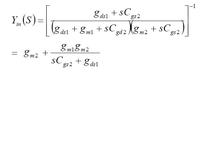I have to agree that using the = sign in the above equation is quite a stretch, as threekingtiger points out you can fully expand the expressions and see what's to be tossed (and assumed!) for that equality to even hold as an approximation.

The approximations required are the usual for saturated FETs and low frequency:
gm1>>gds1 (saturation)
Cgs2>>Cgd2 (saturation)
ignore terms in s^2 (low frequency)

Plus to get there you need to assume gm1~gm2... very rough math

Now the method and the result are quite unorthodox (but somewhat ingenious) because one would usually proceed differently and write the expression as
Y(s)= Y0 (1+s/z1)(1+s/z2)/(1+s/p1)
things are a lot more clear-cut that way:
- you make the saturation approximations I mentioned above (gm+gds ~ gm)
- you toss the s/z2 because (apparently) you are not interested in high frequencies, |s|<< z2 ~ gm1/Cgd2
- you are left with
Y(s) ~ Y0 (1+s/z1)/(1+s/p1)
Y0 = gm1 gm2 / gds1
z1 ~ gm2/Cgs2
p1 = gds1/Cgs2

if you are interested in even lower frequencies than you notice that p1<<z1 so you neglect s/z1 and you can write
Y(s) ~ Y0 / (1+s/p1)

It looks a lot more straightforward this way...

•Points: 2
I did some suggests of you but the operation frequency isnt very low and is in the GHz range, if its possible for you see this thesis from below link page: 89 - 92 and tell me your idea

apparently you were onto something because now equation 5.7 in that thesis has a different result - try downloading a new copy of it.

In any case once you have a rational function in the form
Y(s)= Y0 (1+s/z1)(1+s/z2)/(1+s/p1)
Y0 = gm1 gm2 / gds1
z1 ~ gm2/Cgs2
z2 ~ gm1/Cgd2
p1 = gds1/Cgs2
under the assumption of saturation and gm1~gm2 then you can always shift your approximation to different frequency ranges
Notice that
p1<<z1<<z2

So let's say you are trying to look at the frequency behavior around z1 then
1+s/p1 ~ s/p1
1+s/z2 ~ 1
and you are left with
Y ~ Y0 p1 (1+s/z1)/s = Y0 p1 / s + Y0 p1 / z1 = 1/(s Leq) + Geq
where you can easily identify an inductive term and a resistive term (in parallel)
Leq = 1/(Y0 p1) = Cgs2 / gm1 gm2
Geq = Y0 p1 /z1 ~ gm1

Now what he is trying to do is to model this over a wider (possibly the entire) frequency range... you have two options: you assume his equivalent circuit is correct and calculate its Y and compare it to the one above OR more skeptically (one would not be surprised this new result is messed up as well) you expand the expression above to get something in the form
Y(s)= B (z1+s)(z2+s)/(p1+s) = B (z1-p1+(p1+s))(z2+s)/(p1+s)= B [ (z2+s) + (z1-p1)(z2+s)/(p1+s) ] =
= B [ (z2+s) + (z1-p1)(z2-p1+(p1+s))/(p1+s) ] = B [ (z2+s) + (z1-p1) + (z1-p1)(z2-p1)/(p1+s)]
where [assuming I did not make a mistake] you can easily identify a parallel combination of admittances
-) Ceq = B = Y0 p1 / (z1 z2) ~ Cgd2
-) Geq = B(z1+z2-p1) ~ B z2 ~ gm1
-) a third term
~ B z1 z2 / (p1 + s)
which can be written as the admittance of the series of one Req and one Leq : 1/R 1/sL / (1/sL +1/R) = 1/L / (s + R/L)
Leq = 1 / (B z1 z2) = 1 / (Y0 p1) = Cgs2 / gm1 gm2
Req = p1 / (B z1 z2) = 1/ Y0 = gds1/gm1 gm2

In summary the new expression 5.7 is still wrong
Of course I am assuming the initial form was correct (which I very much doubt now) and that I did not make a mistake in my calculation (very much possible)

I will double check later, you should do the same---------- Post added at 12:01 ---------- Previous post was at 10:45 ----------

It looks like our author (or myself - since I have not rechecked my math) was caught in a moment of mathematical weakness; I am getting from his circuit
Y(s) = s Cgs1 + (gm2 + s Cgs2) / (gds1 + s Cgs2)
which gives the same
Leq, Req, Geq
but
Ceq = Cgs1
which makes a lot more sense

____________
There is an error in this last expression for Y(s) see next post for the correct result

Last edited:

Hi dgani

no I was not onto something else, I read this thesis and the equation that I attached above is 5.7 but I deleted gds2 and SCgs1 because these are parallel admytances with Zin(S) in 5.6 ,if you attention, you can see these parameters arent in Zin(S) and then how it is possible to see them in the inverse of Zin(S) !!!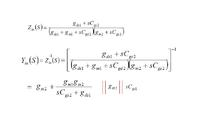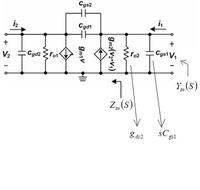Last edited:

'being onto something' means having found something interesting, specifically you have found a mistake in that thesis... now I don't have my back of the envelope sketch in front of of me but your assumption that (5.7) is not the inverse of (5.6) might be correct, this might be one of the mistakes he makes; on the other hand in the final result I get gm1 in place of his gm2 as Geq (gds2 is negligible)... let's try here:

-v2(gds1+sCgd2)-gm1 v1+ s Cgs2 (v1 -v2) =0
i1 - s Cgs2 (v1 -v2) + gm2(v2 - v1) -v1(gds2+sCgs1)=0

|(-gm1+sCgs2) -(gds1+s(Cgd2+Cgs2)) | |v1| = | 0 |
|-(gm2+gds2+s(Cgs2+Cgs1)) gm2+s Cgs2 | |v2| |-i1|

|(-gm1+sCgs2) -(gds1+sCgs2) | |v1| = | 0 |
|-(gm2+s(Cgs2+Cgs1)) gm2+s Cgs2 | |v2| |-i1|

-i1(gds1+s Cgs2)
v1= -------------------------------------------------------------
[(-gm1+sCgs2)(gm2+s Cgs2)-((gds1+sCgs2)(gm2+s(Cgs2+Cgs1))]

Y(s)= gm2+s(Cgs2+Cgs1) + (gm1-sCgs2)(gm2+s Cgs2) / (gds1+s Cgs2)

which looks already quite different than his result; the last term can be written in the form
Y3= B (z1-s)(z2+s)/(p1+s) = B (z1-s)(z2-p1+p1+s)/(p1+s) =
= B [ (z2-p1) (z1-s)/(p1+s) +(z1-s) ] =
= B [ (z2-p1) (z1+p1-p1-s)/(p1+s) +(z1-s) ] =
= B [ (z2-p1) (z1+p1)/(p1+s) -(z2-p1) +(z1-s) ]
where
B=Cgs2, z1=gm1/Cgs2, z2=gm2/Cgs2, p1=gds1/Cgs2
hence p1<<z1, z2

Y3 ~ B [ z2 z1/(p1+s) -z2 +z1-s ] = gm1 -gm2 -s Cgs2 + gm1 gm2 / (gds1+s Cgs2)

that placed in Y gives

Y(s)= gm1+ s Cgs1 + gm1 gm2 / (gds1+s Cgs2)

so you can see his result is incorrect (gm2 instead of gm1) and inconsistent (he keeps gds2 that was negligible during his calculation)

Hopefully this is clear enough

•Points: 2

### hsh22

Points: 2
Hi dgani

thanks for your complete answer to my question, its so clear and sorry for my weak english language
I think the author of the thesis for calculation of 5.5 and 5.6 did not consider ro2 and Cgs1 and first calculate Zin(s) that is shown in the picture I have attached and after calculation of Zin(s) and inverse it, added them to final admytance equation (5.7) what is your idea about this?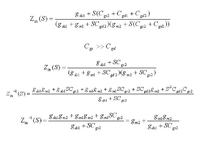sorry my post sounded offensive to you initially, glad we cleared that out

As of your equations, notice that even if you want to consider the small signal analysis of only that part of the circuit (w/o ro2 and Cgs1), the final result should still be coherent with what I posted, you should end up with
Y(s)= gm1+ gm1 gm2 / (gds1+s Cgs2)
getting gm2 instead of gm1 (even though not completely off in terms of order of magnitude) is still indicative of excessive approximation -there is a factor of 2 between gm1 and gm2 in following sections of the thesis so that would be an unnecessary large approximation

I did not check your initial expression (I will later) matches the new circuit but it seems suspicious to me that
- the first factor on the denominator includes Cgd2 by itself as in the complete circuit impedance it only appear in parallel to Cgs2 and so it can be neglected
- you get no negative sign, which should results from the presence of the miller cap Cgs2

Also
- assuming Cgs>>Cgd w/o assuming gm>>gds is hard to justify as it is hard to have one w/o the other in practice, you should get rid of it in gm1+gds1 before expanding the expression
- neglecting the term in s^2 is hard to justify as we (you made this clear only after the initial few posts) are working with RF and are after a full-range model

Hi dgnani

I try to do your calculation at #8 answer, but I cant understand how you write (2) from (1) :sad: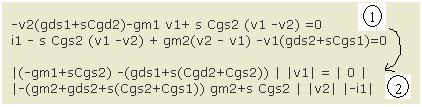Hi dgnani

I try to do your calculation at #8 answer, but I cant understand how you write (2) from (1) :sad:(2) is (1) written with matrices, just hard to make matrices with text, plus multiple spaces are stripped from your formatting so the result is hard to understand.
Here is (2) written as a linear system of equations:
(-gm1+sCgs2) v1 -(gds1+s(Cgd2+Cgs2)) v2 = 0
-(gm2+gds2+s(Cgs2+Cgs1)) v1 + (gm2+s Cgs2) v2 = -i1
without the clumsy attempt to matrix notation
I guess I will have to learn how LATEX works in this forum...

•Points: 2
Last edited:

•Points: 2
oh sorry I wrote wrongI mean Y(S)
ok then with this 2 assumption I can drive your final Y(S) equation, now Is this Y(S) valid for all range of frequency or it is valid just for low frequency?

oh sorry I wrote wrongI mean Y(S)
ok then with this 2 assumption I can drive your final Y(S) equation, now Is this Y(S) valid for all range of frequency or it is valid just for low frequency?

yes those are the only two assumptions you need (they should be equivalent specifying saturation as an operating region -unless you go to an extreme deep submicron process...) to derive the final equation for Y(s) valid over the whole frequency range

I use 0.18um for simulation, do you mean for this type of technology Y(S) is valid just for low frequency?

(by extreme deep submicron I mean 65nm and below, you are safe at .180)

The approximations I gave you work for saturation and at all frequencies for a 0.18um process, where are you getting the impression I am saying anything about low frequencies?

from (5.13) at this thesis or you calculate the Q from the Y(S) that you drived with above two assumption, it seems that Q is proportional to Cgs2 but I see the picture that I attached here at a IEEE conf paper (of course has referenced to other journal papers) that shows Q is proportional to inverse of Cgs2, why is it?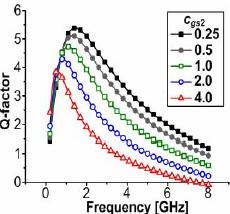its the IEEE conf paper:
IEEE Xplore - Novel High-Q Inductor using Active Inductor Structure and Feedback Parallel Resonance Circuit

I had a quick look at the paper and I am under an even stronger impression that these people are -let's put it that way- not exactly accurate

The thesis was very inconsistent from a mathematical point of view and even in the article he states he needs current i2 to calculate the impedance seen on the active inductor input which is clearly not true.

Then in the paper they come up with this mysterious expression that they simulate instead of running spice!? I think your best option would be to actually run spice on a behavioral model and see what happens

Clearly their plots seem to have nothing to do with the small signal model they use: e.g.
- the natural frequency does not depend on Cgs1
- Q factor decreases with Cgs2
and probably more stuff

As far as I can tell the mistake in on their side but you can verify putting their small signal model in spice and running it

Status
Not open for further replies.